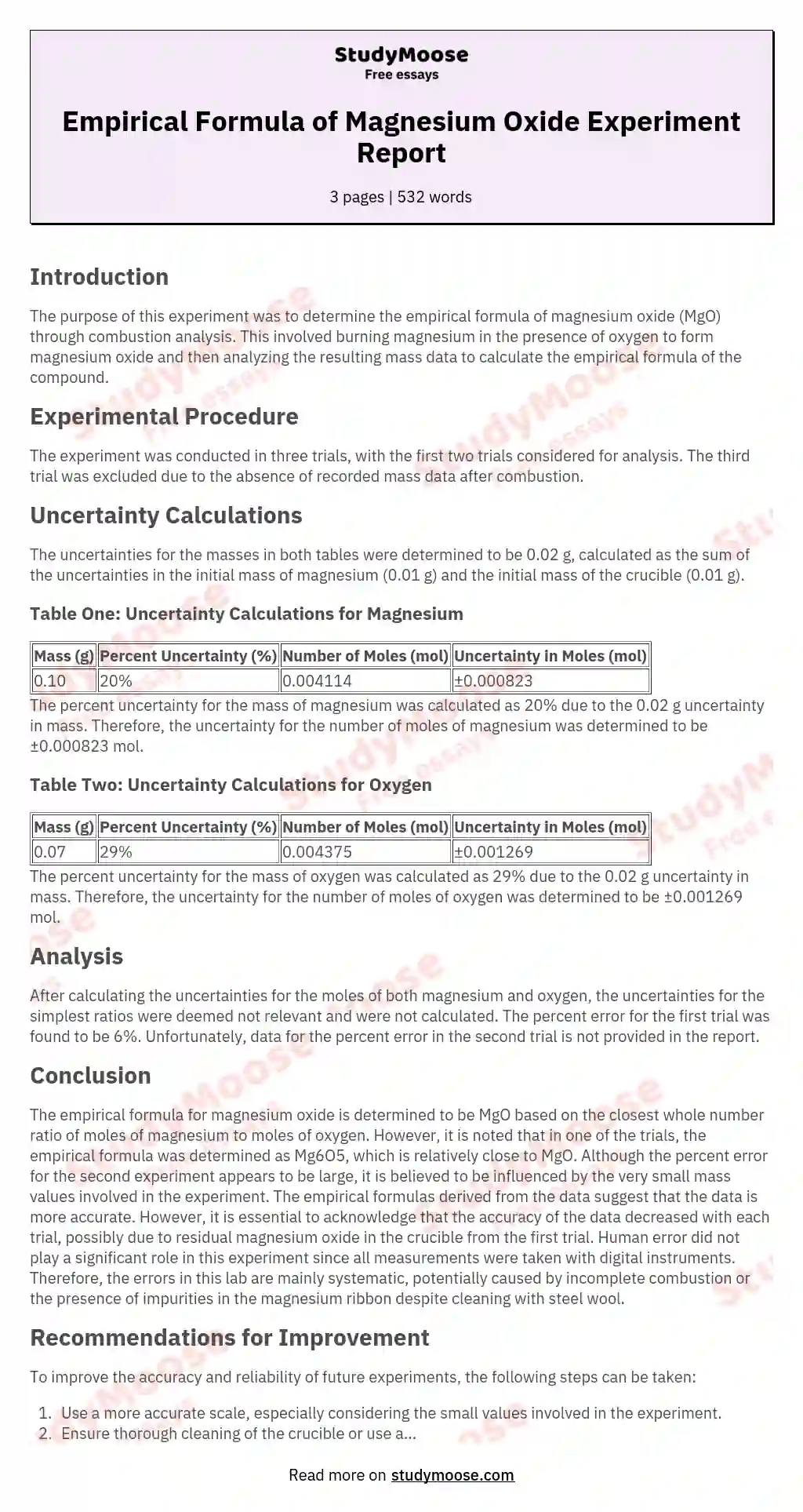# Determining the empirical formula of magnesium oxide lab

Categories: Science

Since multiplying the ratio by five gives the closest values to whole numbers, therefore the empirical formula of the oxide is Mg6O5.

The third trial was not taken into account because the mass after combustion was not recorded.

Uncertainty calculations:

The uncertainties for masses in both tables will be: 0.01+0.01=0.02g

Table one:

Here are the calculations for the uncertainty for the number of moles of magnesium: 0.02g/.10gï¿½100%=20% (the uncertainty for the molecular mass is taken as zero) Therefore the percent uncertainty for the mass of the magnesium is 20%.

0.2ï¿½0.004114=0.000823 This means that the uncertainty for the number of moles in the magnesium is ï¿½0.008228mol.

These are the calculations for determining the uncertainty of the number of moles of oxygen. 0.02g/0.07gï¿½100%=29%.Therefore the percent uncertainty for the mass of the oxygen is 29%

0.29ï¿½0.004375=0.001269. This means that the uncertainty for the number of moles of oxygen is ï¿½0.001269mol.

Table two;

After calculations for uncertainties for the moles of both elements were made, the uncertainty for the moles of magnesium was ï¿½0.

Get quality help nowwriter-CharlotteVerified writer

Proficient in: Science4.7 (348)

“ Amazing as always, gave her a week to finish a big assignment and came through way ahead of time. ”+84 relevant experts are online

000823mol and the uncertainty for oxygen ï¿½0.001269mol

Since the uncertainties for the simplest ratios are not relevant, they were not calculated.

The percent error for the first trial is: 6%

The percent error for the second trial is:

Conclusion:

The empirical formula for magnesium oxide is MgO1.The empirical formula for one of the trials for this lab had this, but the other Mg6O5, which is relatively close.

Even though the percent error for the second experiment seems large, I believe this is due to the fact that very small mass values were being worked with, as well as the fact that the percent error could only be calculated through the ratio, whereas the empirical formulas seem to indicate that the data is more accurate.

Get to Know The Price Estimate For Your Paper
Topic
Number of pages
Email Invalid email

You won’t be charged yet!

The accuracy of the data did however decrease with each trial and I believe this is due to left over magnesium oxide residue in the crucible from the first trial. Besides the third trial in which the crucible was broken, and the possibility of left over oxide from trial one, in trial two, human error does not play a huge role in this lab, because all measurements were taken with a digital instrument.

Therefore the errors in this lab are mainly systematic, but could also be due to an incomplete reaction occurring due to impartial combustion. The precision is distorted by the left over magnesium oxide, the only experimental change between trials one and two. The precision of the scale is very important to the lab, because this is the main source of error. The percent uncertainties were high, but this most likely is due to the fact that very small values were worked with. Overall the charts above in the processing section were thought to be the most efficient and organized ways of expressing the calculations, but the uncertainties were done separately as to not be too messy.

There are a few ways to improve and avoid the above errors. It is unlikely that a more accurate scale could be used but since the experiment works with such small values, this would definitely be more appropriate. Also it would be best to use another crucible or completely clean out the original in order to avoid the error from the second trial. I think the experiment should be completely more times to make more accurate assumptions as to whether or not how well the crucible was cleaned after the initial trial had an effect on the second.

There is a very slight possibility that incomplete combustion did not occur, but increase the accuracy of the experiment it would be best to expose the magnesium as much as possible to oxygen. There may also be impurities in the magnesium ribbon, despite the fact that it was cleaned with steel wool. If making sure the sample was purely magnesium that should be taken into account as well.

1 (TrueKnowledge, 2011)http://www.trueknowledge.com/q/what_is_the_chemical_formula_for_%22magnesium_oxide%22)